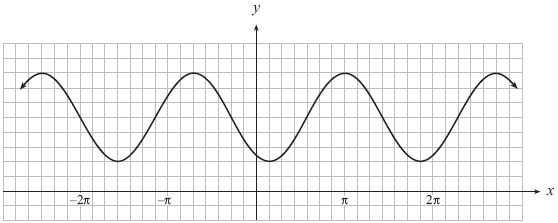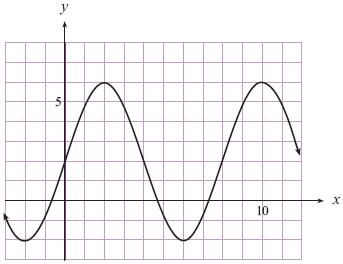# Determining trigonometric functions given their graphs

### Determining trigonometric functions given their graphs

#### Lessons

• 1.
For the following graph, write an equation (with the smallest phase shift) in the form of:
(i) y = a sin b(x - c) + d
(ii) y = - a sin b(x - c) + d
(iii) y = a cos b(x - c) + d
(iv) y = - a cos b(x - c) + d
a)b)• 2.
A sinusoidal curve has a minimum point at $\left( { - \frac{\pi }{3},\; - 5} \right)$ and the closest maximum point to the right is $\left( {\;\frac{\pi }{6},\;3} \right)$. Determine an equation of this curve.